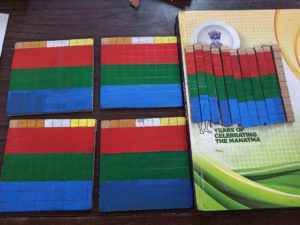# Divisibility Rule by 3?

~Sandhiya, Ganesh Shelke

We, as a team of two, worked on creating the model to demonstrate the divisibility by 3 rule for 3 digit numbers so that students can actually see and visualize the concept.

The basic concept is as follows:

Let’s take the number e.g.  498

The Theory:

To see if it is divisible by 3 or not, we will simplify the number as follows:

First, we will simplify it as Hundred’s place, ten’s place, and one’s placed by splitting these places into 1 + remaining term (9, 99, 999, …)

498 = 4(1+99) + 9(1+9) + 8

Simplifying brackets:

498 = 4 + 4*99 + 9 + 9*9 + 8

Rearranging the terms:

498 = 4(99) + 9(9) + 4 + 9 + 8  (Observe that we get original number back 498)

So, we have 9, 99, 999, … that is completely divisible by 3 and if we multiply these numbers by any number, then also it will be completely divisible by 3. And we will add remaining numbers (4+9+8=23) We put these numbers and divide them as complete blocks of 3 squares. If all blocks are complete, then the number is divisible by 3, otherwise, it’s not. Here in our case, it’s not divisible by 3

Demonstration:To demonstrate this, we have created squares of 100 i.e. 10*10 and 10 i.e. (1*10) and painted every 3 squares in the same color (this demonstrates that it is completely divisible by 3) and then we have one square left from every 1000, 100, 10 blocks. We will add these blank squares, count them and see if we get all complete blocks of 3 squares or not. If we get all the complete blocks of 3 squares, then the number is completely divisible by 3 otherwise it’s not.